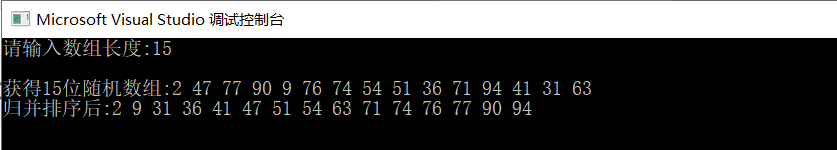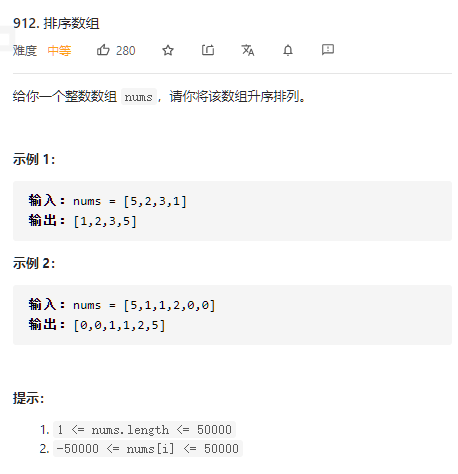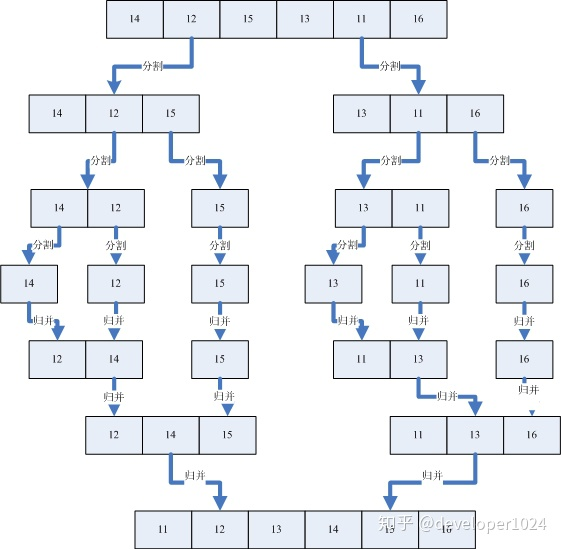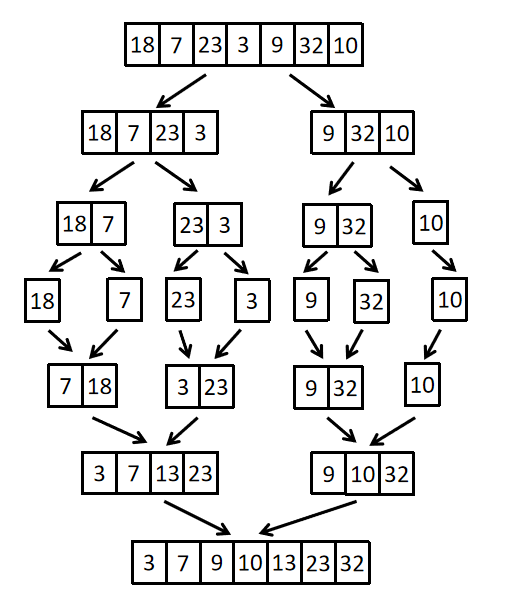• 2022-05-06 16:59:09

本篇文章参考《大话数据结构》一书

归并排序（Merge Sort）是利用归并的思想实现的排序方法，该算法采用经典的分治（divide-and-conquer）策略将问题分成一些小的问题然后递归求解，即分而治之。

#include<iostream>
using namespace std;
#include<vector>

#define initial_capacity 10
//归并排序
class Solution
{
public:

vector<int> merge_sort(vector<int>& ans)
{
vector<int>res;
res.resize(initial_capacity); //需要预先设置起始容量，防止越界
int s = 0, t = ans.size() - 1;
mySort(ans, res, s, t);
return res;
}

void mySort(vector<int>& ans, vector<int>& res, int s, int t)
{
vector<int>temp;
temp.resize(initial_capacity);
if (s == t)
res[s] = ans[s];
else
{
int m = (s + t) / 2;          //将ans数组平分为两个数组
mySort(ans, temp, s, m);      //递归地将前数组归并为有序的temp数组
mySort(ans, temp, m + 1, t);  //递归地将后数组归并为有序的temp数组
myMerge(temp, res, s, m, t);  //将前两个数组归并为res有序数组
}
}

void myMerge(vector<int>& ans, vector<int>& result, int i, int m, int n)
//m,n分别为中间位置以及尾部位置
{
int j, k, l;
for (j = m + 1, k = i; i <= m && j <= n; k++)
{
if (ans[i] < ans[j])
{
result[k] = ans[i++];
}
else
{
result[k] = ans[j++];
}
}

//如果此时前数组未结束，而后数组结束时，将前数组剩余内容依次追加到结果数组
if (i <= m)
{
for (l = 0; l <= m - i; l++)
{
result[k + l] = ans[i + l];
}
}

//与上述反之
if (j <= n)
{
for (l = 0; l <= n - j; l++)
{
result[k + l] = ans[j + l];
}
}

}
};

//打印输出
void myPrint(vector<int>& ans)
{
for (int i = 0; i < ans.size(); i++)
{
cout << ans[i] << "  ";
}
cout << endl;
}

int main()
{
vector<int>ans = { 1,12,3,9,5,4,0,1,8,7 };

Solution s;
auto a = s.merge_sort(ans);
myPrint(a);      //打印输出

system("pause");
return 0;
}算法
更多相关内容
• 主要为大家详细介绍了C++实现归并排序，文中示例代码介绍的非常详细，具有一定的参考价值，感兴趣的小伙伴们可以参考一下
• 6.归并排序归并排序的基本思想代码实现适用说明 归并排序的基本思想 1.基本思想（递归）： 思路来源：两个有序序列，按序合并只需遍历一趟，效率较高； 归并排序：利用分-治-合的思想，先将待排序列划分为子序列，...

# 归并排序的基本思想

1.基本思想（递归）：

• 思路来源：两个有序序列，按序合并只需遍历一趟，效率较高；
• 归并排序：利用分-治-合的思想，先将待排序列划分为子序列，待子序列有序（元素个数为1）后，再将子序列两两合并，从而得到完整有序的序列。2.算法步骤：

1. 申请空间，分配一个与待排数组大小相同的数组；
2. 利用递归函数，由中间位置不断划分序列，直到元素个数为1；（递去）
3. 子序列按照升(降)序两两合并；（归来）

# 代码实现

#include "pch.h"
#include <iostream>
#include <time.h>
#include <algorithm>
using namespace std;

/*排序方法类*/
class MySort {
public:
int n;
int* A;
//构造函数 ，便于挑选不同的排序方法
MySort(int N) {
this->n = N;
A = new int[this->n];
this->SetArray();
}
//随机初始化数组
void SetArray() {
srand(time(0));
for (int i = 0; i < n; i++) {
A[i] = rand() % 100 + 1;
}
}
//打印数组
void Print() {
for (int i = 0; i < n; i++) {
cout << A[i] << " ";
}
cout << endl;
}

//归并排序
void merge_sort(int *A, int n){
if (n < 2) return;
int *arrtmp = new int[n];         //分配一个与待排序数组大小相同的数组
_merge_sort(A, arrtmp, 0, n - 1);  //调用递归函数进行排序
delete[] arrtmp;
}
void _merge_sort(int *A, int *arrtmp, int start, int end) {
if (start >= end) return;             //递归终止条件（元素个数为1）
int mid = start + (end - start) / 2;    //以下5行，将数组划分为2个子数组，分别继续递归
int start1 = start, end1 = mid;
int start2 = mid + 1, end2 = end;
_merge_sort(A, arrtmp, start1, end1);
_merge_sort(A, arrtmp, start2, end2);
int i = start;   //已排序数组arrtmp计数器
while (start1 <= end1 && start2 <= end2) 	//升序合并两个子数组至arrtmp中
arrtmp[i++] = A[start1] < A[start2] ? A[start1++] : A[start2++];
while (start1 <= end1) 	arrtmp[i++] = A[start1++];   //剩余元素直接加入数组arrtmp尾部
while (start2 <= end2)	arrtmp[i++] = A[start2++];
memcpy(A + start, arrtmp + start, (end - start + 1) * sizeof(int));  //将已排序数组arrtmp复制回到A中，排序完成
}

};

int main(){
//初始化数组
int N;
cout << "请输入数组长度:";
cin >> N;
MySort sort(N);   //构造排序方法类
cout << endl;
cout << "获得"<<N<<"位随机数组:" ;
sort.Print();
sort.merge_sort(sort.A, N);
cout << "归并排序后:";
sort.Print();
cout << endl;
return 0;
}


代码验证：# 适用说明

• 归并排序是建立在归并操作上的一种有效、稳定的排序算法，时间复杂度为O(nlogn)，归并排序时需要和待排序记录个数相等的存储空间，所以空间复杂度为 O(n)。
• 归并排序适用于数据量大，并且对稳定性有要求的场景。
展开全文• 八大排序之归并排序C++详解题目链接思路分析解题代码 题目链接 这道题目链接是用来测试我们所写算法的正确性 排序数组 思路分析 我们想利用这道题目来讲解归并排序，首先我们来看一下归并排序的定义 归并排序，是...

# 题目链接

这道题目链接是用来测试我们所写算法的正确性

排序数组## 思路分析

我们想利用这道题目来讲解归并排序，首先我们来看一下归并排序的定义

归并排序，是创建在归并操作上的一种有效的排序算法。算法是采用分治法（Divide and Conquer）的一个非常典型的应用，且各层分治递归可以同时进行。归并排序思路简单，速度仅次于快速排序，为稳定排序算法，一般用于对总体无序，但是各子项相对有序的数列。

基本思想
归并排序是用分治思想，分治模式在每一层递归上有三个步骤：

• 分解（Divide）：将n个元素分成个含n/2个元素的子序列。
• 解决（Conquer）：用合并排序法对两个子序列递归的排序。
• 合并（Combine）：合并两个已排序的子序列已得到排序结果。

对于分解来说，我们就是需要将数组内的数字不断的划分，直到划分到最小区间，当划分到最小区间之后，我们就需要开始归并的过程，此时需要注意的是我们需要借助额外的数组来存储我们归并过程中的有序子数组
示例图如下具体的我们以一组无序数列｛14，12，15，13，11，16｝为例分解说明，如下图所示：### 解题代码

class Solution {
public:
void mergeSort(vector<int>& nums, int left, int right, vector<int>& tmp)
{
//此时数组内数字都为单个，开始进行归并
if(left >= right)
return ;
//此时子数组无序，需要继续分割
int mid = left + (right - left)/2;
mergeSort(nums,left, mid, tmp);
mergeSort(nums, mid+1, right, tmp);

//开始归并
//确定辅助数组起始位置
int index = left;
//此时某一小区间为[1, 3] [2, 4];
int left1 = left;
int right1 = mid;
int left2 = mid+1;
int right2 = right;

while(left1 <= right1 && left2 <= right2)
{
if(nums[left1] < nums[left2])
{
tmp[index++] = nums[left1++];
}
else
{
tmp[index++] = nums[left2++];
}
}
//当循环退出时，说明某一小区间归并完毕，此时要将其后的空间添加到辅助数组
while(left1 <= right1)
{
tmp[index++] = nums[left1++];
}

while(left2 <= right2)
{
tmp[index++] = nums[left2++];
}
//处理前 [1,3] [2, 4]
//处理后tmp  [1,2,3,4]
//拷贝至nums
//将子区间处理完毕，现在处理原来数组
for(int i = left; i <= right; ++i)
{
nums[i] = tmp[i];
}
}
vector<int> sortArray(vector<int>& nums) {
vector<int> tmp(nums.size());
mergeSort(nums, 0, nums.size()-1, tmp);
return nums;
}
};


### 进阶指南

此处可以跳转至八大排序之快速排序C++详解

展开全文• 归并排序（Merge sort） 排序思想：将初始序列的n个元素看成n个有序的子序列，每个子序列中只有一个元素，将其两两归并，得到n/2个长度为2(或1、子序列不为偶数则有落单)的有序子序列，再两两归并…以此类推直到...

归并排序（Merge sort）

1. 排序思想：将初始序列的n个元素看成n个有序的子序列，每个子序列中只有一个元素，将其两两归并，得到n/2个长度为2(或1、子序列不为偶数则有落单)的有序子序列，再两两归并…以此类推直到得到n长的有序序列。
2. 归并思想：两个子序列，分别有一个指针指向其首部，指针指向的元素进行对比，小的放入辅助数组里，指针后移，大的不动，直到两两对比完成，此时如果有某一子序列中的元素并没有对比完，则直接放入辅助数组。3. 优缺点：效率高且稳定，但是消耗的辅助空间与原数据空间成正比。
4. 复杂度：时间复杂度O(nlogn)
空间复杂度O(n)
5. 稳定性：稳定

源代码

/**************************
题目：归并排序
划分成很小的组，然后两两归并
***************************/
#include<iostream>
using namespace std;

void Merge(int[], int, int[], int, int, int)  //归并函数的声明【把归并函数提到该函数前面，则不用声明】
//归并排序
//参数：
//		numbers[]：原数组
//		length：数组元素的个数（数组长度）
//		temp[]：辅助数组
//		begin：数组开头的下标
//		end：数组结尾的下标
void MergeSort(int numbers[], int length, int temp[], int begin, int end)
{
//1. 同样判断传入的参数是否有效
if (numbers == nullptr || length <= 0 || begin < 0 || end >= length)
throw new exception("Invalid input.");

//2. 作为递归的结束条件，开始下标和结束下标相等时，说明子序列中只有一个元素，看作有序的
if (end - begin == 0)
return;

//3. 定义中间变量，将数组分半【如果有7个元素，下标0-6，则middle=3，数组分为长度为4和3的两段】
int middle = ((end - begin) / 2 ) + begin;
//4. 递归，先递归左半边，再递归右半边，将左右子序列不断分为长度为1的子序列才停止递归
MergeSort(numbers, length, temp, begin, middle);
MergeSort(numbers, length, temp, middle + 1, end);
//5. 再慢慢归并
Merge(numbers, length, temp, begin, end, middle);
}
//归并函数
//参数：
//		numbers[]：原数组
//		length：数组元素的个数（数组长度）
//		temp[]：辅助数组
//		begin：数组开头的下标
//		end：数组结尾的下标
//		middle：数组中间的下标
void Merge(int numbers[], int length, int temp[], int begin, int end, int middle)
{
//1. 判断是否有不符合要求的参数传入，有则抛出错误
if (numbers == nullptr || length <= 0 || begin < 0 || end >= length)
throw new exception("Invalid input.");

//2. 将原序列从中分开
int leftIndex = begin;		//左边序列的开始（左边序列的结尾是middle）
int rightIndex = middle + 1;//右边序列的开始（右边序列的结尾是end）
int tempIndex = begin;		//辅助数组的下标
//3. 当左右子序列尚未到头时，循环
while (leftIndex <= middle && rightIndex <= end)
{
//4. 两两对比判断，谁大谁就放入辅助数组，同时指针后移
if (numbers[leftIndex] < numbers[rightIndex])
temp[tempIndex] = numbers[leftIndex++];
else
temp[tempIndex] = numbers[rightIndex++];
//5. 辅助数组下标++
++tempIndex;
}

//6. 当左边或右边子序列尚未到头时，直接放入辅助数组
while (leftIndex <= middle)
temp[tempIndex++] = numbers[leftIndex++];

while (rightIndex <= end)
temp[tempIndex++] = numbers[rightIndex++];

//7. 再将辅助数组中已经有序的元素覆盖掉原数组中无序的元素，使原数组变成部分有序
for (int i = begin; i <= end; ++i)
numbers[i] = temp[i];
}
//简单测试
int main(int arvc, char* argv[])
{
const int length = 9;
int nums[length] = { 18, 7, 23, 3, 9, 32, 10 , 99, 0};
int *temp = new int[length];

MergeSort(nums, length, temp, 0, 8);

for (int i = 0; i < length; i++)
cout << nums[i] << "  ";

delete[] temp;
temp = nullptr;
cout << endl;
return 0;
}


仍有不足，欢迎交流。

展开全文算法 数据结构
• 主要介绍了C++实现自底向上的归并排序算法,结合实例形式较为详细的分析总结了自底向上的归并排序算法的原理与具体实现技巧,需要的朋友可以参考下
• 基于visual studio2010，的程序开发，...............................................................................................sort
• 课程的随堂作业，C语言的，用dev就能运行，萌新代码，勿喷，仅仅帮助不想写作业的朋友方便一下，反正老师也不会仔细检查的
• ## 归并排序C++算法实现

万次阅读 多人点赞 2019-03-31 15:41:17
定义：归并排序（MERGE-SORT）是建立在归并操作上的一种有效的排序算法,该算法是采用分治法（Divide and Conquer）的一个非常典型的应用。将已有序的子序列合并，得到完全有序的序列；即先使每个子序列有序，再使子...
• 二路归并排序 基本思想 二路归并排序就是将两个有序子表归并成一个有序表。首先我们得有一个算法用于归并：两个有序表放在同一数组的相邻位置上，arr[left]到arr[center-1]为第一个有序表，arr[center]到arr[right...排序算法
• #include &lt;iostream&gt;#include &lt;vector&gt;#include &lt;algorithm&gt;using namespace std;class Solution{public: void guibing(vector&lt;...2) retur...
• P话不多说，上代码main.cppresultnotice main.cpp #include <random> #include <chrono> #include <...// 排序数据大小 constexpr int n = 10000000; // 最大并发单位实际数量 static atomi多线程 算法 thread
• 使用C++书写的归并排序算法，希望对各位有用。也请大牛指教代码中有何不足的地方！
• 归并排序遵循分治法的思想：将原问题分解为几个规模较小但类似于原问题的子问题，递归地求解这些子问题，然后再合并这些子问题的解来建立原问题的解。分治模式在每层递归时都有三个步骤：分解、解决、合并。归并排序...
• 对左半部分[low,mid]递归地进行归并排序 //3.对右半部分[mid+1,high]递归地进行归并排序 //4.将左右两个有序子序列Merge为一个 //归并排序 const int N = 1000; int* B = (int*)malloc(N * sizeof(int));//辅助数组B...算法
• ## 归并排序c++实现

千次阅读 2015-09-14 19:54:06
#include using namespace std;...*归并算法 *将数组a中的下标s~m 和m~e按从小到大的顺序合并 */ void Merge(int *a,int s,int m,int e) { int n1,n2; n1=m-s+1; n2=e-m; int *l=new int[n1+1]; int *h=n
• 将n个子数列两两排序，得到n/2个有序的分别有两个元素的数列 将n/2个子数列再两两排序，得到n/4个有序的子数列。 重复上述步骤，最终得到一个有序数列 算法实现（递归实现） #include<iostream> #include<...数据结构与算法
• ## 归并排序—C++实现

千次阅读 2020-05-16 14:36:31
相比于快速排序，归并排序就十分稳定，无论是最好还是最差的情况，它的时间复杂度都为O（nlogn）。归并排序同样利用了递归的思想，它的思想是把一...下面我们来看看C++如何实现归并排序 归并排序代码 #include<iost算法 数据结构
• 文章目录归并排序实例演示代码实现结果展示归并排序的特性总结： 归并排序 基本思想： 归并排序（MERGE-SORT）是建立在归并操作上的一种有效的排序算法,该算法是采用分治法的一个非常典型的应用。将已有序的子序列...算法 数据结构 排序算法
• 本文主要是针对归并排序进行分析，分别从递归的角度和分治的角度对归并进行认识，并附上c++的实现 一、归并排序介绍（代码在大标题二） 同样，这里我们以一个乱序数组为例。有数组 a a a a a a...
• } 归并排序算法模板： void merge_sort(int q[], int l, int r) { if (l >= r) return; int mid = l + r >> 1; merge_sort(q, l, mid); merge_sort(q, mid + 1, r); int k = 0, i = l, j = mid + 1; while (i ) if ...算法
• 快速排序为先分隔在左右排序，归并排序为先左右排序再合并 #include <vector> #include <stdlib.h> using namespace std; class Solution { public: //临时数组 vector<int> tempArr; ...算法
• ## C++链表归并排序

千次阅读 2022-04-20 19:29:22
你需要先会写数组的归并排序。 除此之外，你还要对递归的过程有很清晰的认识。 你需要会用熟练的使用指针，这是C++玩家必备技能。 代码里的List，可能命名成Node更加合适，我也不知道我当时这么想的...算法...

# 归并排序c++c++ 订阅